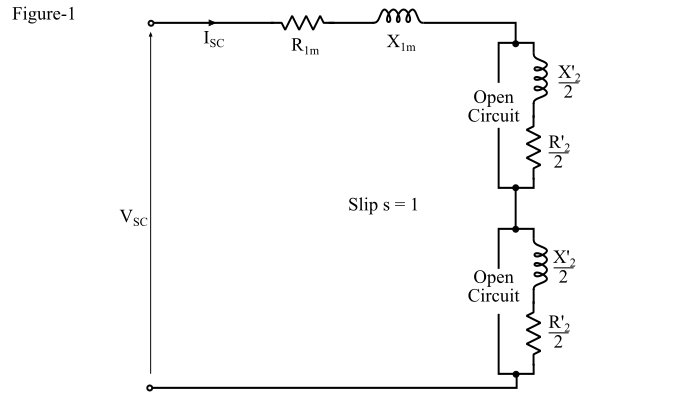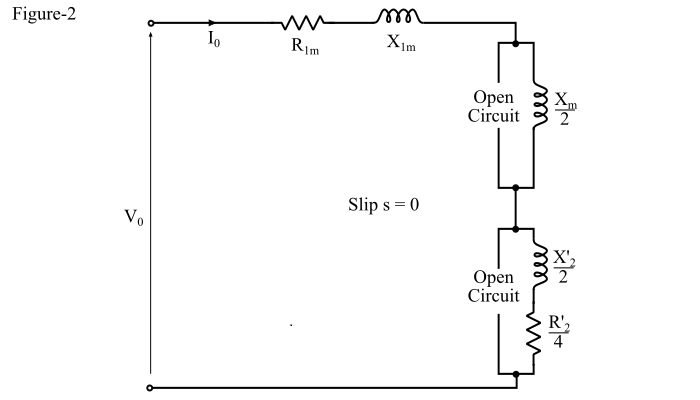# No-Load Test and Blocked-Rotor Test on Single-phase Induction Motor

The equivalent circuit parameters of a single-phase induction motor can be determined from the blocked rotor and no-load tests. Except, the capacitor-run motor, the blocked rotor and no-load tests are performed with auxiliary winding kept open.

## Blocked Rotor Test on Single-phase Induction Motor

In the blocked rotor test, the rotor of the motor is blocked, i.e., remains at rest. A reduced voltage is applied to the stator so that the rated current flows in the main winding. The voltage, current and power are measured.Vsc, Isc and Psc are the voltage, current and power under the blocked rotor conditions. With the rotor blocked, the slip s = 1, then the magnetising reactance $(\frac{𝑋_{𝑚}}{2})$in the equivalent circuit of the single-phase induction motor is so large compared with impedance $(\frac{{𝑅^{'}}_{2}}{2}+𝑗\frac{{X^{'}}_{2}}{2})$that it may be neglected from the equivalent circuit. Thus, the equivalent of the single-phase induction motor at slip s = 1 reduces to the circuit as shown in Figure-1.

Refer Figure-1. The equivalent impedance referred to the stator is given by,

$$\mathrm{𝑍_{𝑒𝑞} =\frac{𝑉_{sc}}{𝐼_{sc}}… (1)}$$

The equivalent series resistance of the motor is given by,

$$\mathrm{𝑅_{𝑒𝑞} =𝑅_{1𝑚} +\frac{{𝑅^{'}}_{2}}{2}+\frac{{𝑅^{'}}_{2}}{2}=𝑅_{1𝑚} +{𝑅^{'}}_{2}=\frac{𝑃_{sc}}{{𝐼^{2}_{sc}}}… (2)}$$

Where, the R1m is the resistance of the main stator winding which is already measured, then the effective rotor resistance at the line frequency is given by,

$$\mathrm{{𝑅^{'}}_{2}=\frac{𝑃_{sc}}{{𝐼^{2}_{sc}}}-𝑅_{1𝑚} … (3)}$$

The equivalent leakage reactance of the motor is given by,

$$\mathrm{𝑋_{𝑒𝑞} = 𝑋_{1𝑚} +\frac{{X^{'}}_{2}}{2}+\frac{{X^{'}}_{2}}{2}=𝑋_{1𝑚} + {𝑋^{′}}_{2}… (4)}$$

As, the leakage reactances X1m and X’2 cannot be separated out so we make a simplified assumption that both the reactances are equal i.e. $𝑋_{1𝑚} = {𝑋^′}_{2}$.

$$\mathrm{∴ 𝑋_{1𝑚} ={X^{'}}_{2}=\frac{𝑋_{𝑒𝑞}}{2}=\frac{1}{2}×\sqrt{{Z^{2}_{𝑒𝑞}}-{R^{2}_{𝑒𝑞}}}… (5)}$$

Therefore, by performing blocked rotor test on the single-phase induction motor, the parameters of the equivalent circuit can be determined if the resistance R1m is known.

## No-Load Test on Single-phase Induction Motor

In the no-load test of the motor, the motor is run without load at the rated voltage and rated frequency. The voltage, current and power input are measure using voltmeter, ammeter and wattmeter respectively.

At no-load the slip s in the motor is very small (closed to zero) and hence, the impedance $(\frac{{R^{'}}_{2}}{2𝑠})$is very large as compared to the magnetising impedance $(\frac{𝑋_{𝑚}}{2})$.

Also,

$$\mathrm{\frac{{𝑅^′}_{2}}{2(2 − 𝑠)}\cong\frac{{𝑅^′}_{2}}{2(2 − 0)}=\frac{{𝑅^′}_{2}}{4}}$$

Thus, the resistance $[\frac{{𝑅^′}_{2}}{2(2 − 𝑠)}]$ which is associated with the backward rotating magnetic field is very small as compared to the magnetising impedance $(\frac{𝑋_{𝑚}}{2})$, that the backward magnetising current is negligible. Therefore, under the no-load conditions, the equivalent circuit of the single-phase induction motor simplified to that shown in Figure-2.Therefore, the equivalent reactance of the motor at no-load is given by,

$$\mathrm{𝑋_{0𝑒𝑞} = 𝑋_{1𝑚} +\frac{𝑋_{𝑚}}{2}+\frac{{𝑋^′}_ {2}}{2}… (6)}$$

Since the values of the reactances X1m and X’2 are known from the blocked rotor test, the value of magnetising reactance (Xm) can be determined from the equation (6).

If V0, I0 and P0 are the voltage, current and the power input respectively in the no-load test. Then, the power factor of the motor at no-load is given by,

$$\mathrm{cos\:φ_{0} =\frac{𝑃_{0}}{𝑉_{0}𝐼_{0}}… (7)}$$

The equivalent impedance at no-load is given by,

$$\mathrm{𝑍_{0𝑒𝑞} =\frac{𝑉_{0}}{𝐼_{0}}… (8)}$$

The equivalent reactance of the motor at no-load is given by,

$$\mathrm{𝑋_{0𝑒𝑞} = 𝑍_{0𝑒𝑞}\:sin\:φ_{0}}$$